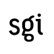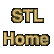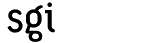# Binary Function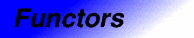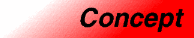Category: functors Component type: concept

### Description

A Binary Function is a kind of function object: an object that is called as if it were an ordinary C++ function. A Binary Function is called with two arguments.

Assignable

### Associated types

 First argument type The type of the Binary Function's first argument. Second argument type The type of the Binary Function's second argument. Result type The type returned when the Binary Function is called

### Notation

 F A type that is a model of BinaryFunction X The first argument type of F Y The second argument type of F Result The result type of F f Object of type F x Object of type X y Object of type Y

### Definitions

The domain of a Binary Function is the set of all ordered pairs (x, y) that are permissible values for its arguments.

The range of a Binary Function is the set of all possible value that it may return.

### Valid expressions

Name Expression Type requirements Return type
Function call f(x,y)   Result

### Expression semantics

Name Expression Precondition Semantics Postcondition
Function call f(x,y) The ordered pair (x,y) is in f's domain Calls f with x and y as arguments, and returns a value of type Result  The return value is in f's range

### Models

• Result (*)(X,Y)

### Notes

 Two different invocations of f may return different results, even if f is called with the same arguments both times. A Binary Function may refer to local state, perform I/O, and so on. The expression f(x,y) is permitted to change f's state.